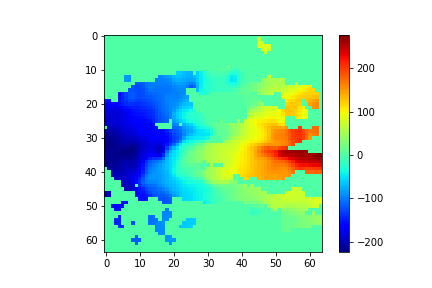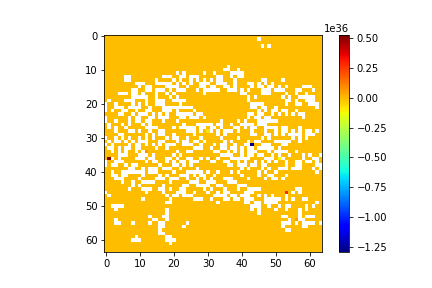# torchvision.Transforms.ToTensor changing scale

I am using `torchvision.Transforms` to prepare images for a network but when I perform the operation I get strange scaling of the image.

Here is an image before transforming which is just a numpy array:and now performing the transform…

``````transform = transforms.Compose([transforms.ToTensor()])
tensor = transform(image.reshape(*image.shape,1)).float().numpy()

plt.figure()
plt.imshow(tensor[0,:,:],cmap='jet')
plt.colorbar()
``````Is this because the ToTensor class expects an image of a certain type or am I missing something in the data maybe?

1 Like

`ToTensor` transforms the image to a tensor with range [0,1].
Thus it already implies some kind of normalization. If you want to use the normalization transform afterwards you should keep in mind that a range of [0,1] usually implies mean and std to be around 0.5 (the real values depend on your data). These are the values you should pass to the normalization transform as mean and std.

Which means you need to have your image in range of [0,255] before. Maybe your loaded image already lies in a range of [0,1]?

1 Like

Thank makes sense but my colorbar has a range of ~1e36 so it doesn’t seem to be normalising it between 0-1 strangely

277.83605046608886 -223.96290534932243

If I divide my images by 255 when they are loaded in, then maybe that will work.

Update: That seems to work! I guess the transformation cannot handle data outside the 255 range

Or you could do

``````img = LOAD_YOUR_IMAGE
img += img.min()
img *= 255/img.max()
img = np.astype(np.uint8)
``````

If your image is a numpy array (which I assume from your plotting code). This would work for all image ranges and would always use the full range of [0,255]. The disadvantage however would be that if you have some effects with high intensity only in part of the images, they would be scaled differently. Another approach would be to calculate mean and std during your datasets init (would take some time for huge dataset)

1 Like

take care of this issue as well.

Would that not be: `img -= img.min()` instead of +?

If I try that I start off with an image:and then get the following image after the transform:strangely with a mean of nan:

``````tensor[0,:,:].mean()

Out: nan
``````

which is odd as I use `tensor[tensor != tensor] = 0` to remove all nan values upon loading the file.

You’re right.

Do you try this before removing NaNs or after?

Can you post your whole transformation code?

To avoid this, I added the conversion to unsigned ints, since ints are expected to be in range [0,255]

Ah I missed the uint8 line as Jupyter Notebooks doesn’t support `np.astype` but I’ve replaced it with `img.astype(np.uint8)` and it works perfectly now.

So for anyone who comes across this thread, here is the complete code:

``````img[img != img] = 0
img -= img.min()
img *= 255/img.max()
img = img.astype(np.uint8)
transform = transforms.Compose([transforms.ToTensor(),transforms.Normalize(, )])
tensor = transform(img.reshape(*img.shape,1)).float()
``````

And the final image after transform:Many thanks as always1 Like